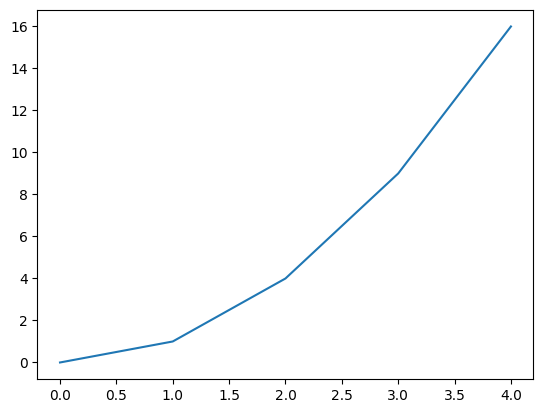# Comprehensions (List, Dictionary, Set)¶

## Intro¶

• Python is expressive

• Clear syntax for everything

• Sometimes a bit short to the point

• ⟶ Comprehensions, consequentially

## Simplest: List Comprehension¶

```l = []
for i in range(5):
l.append(i**2)

l
```
```[0, 1, 4, 9, 16]
```

This is clumsy. Want dedicated sytax!

```[i**2 for i in range(5)]
```
```[0, 1, 4, 9, 16]
```

Even with an included if statement …

```[i**2 for i in range(5) if i%2==0]
```
```[0, 4, 16]
```
```%matplotlib inline
import matplotlib.pyplot as plt

x = list(range(5))
y = [i**2 for i in x]
plt.plot(x, y)
plt.show()
```## Dictionary Comprehension¶

```{i: i**2 for i in range(5)}
```
```{0: 0, 1: 1, 2: 4, 3: 9, 4: 16}
```
```{i: i**2 for i in range(5) if i%2==0}
```
```{0: 0, 2: 4, 4: 16}
```

## Set Comprehension¶

```{i for i in range(5)}
```
```{0, 1, 2, 3, 4}
```
```{i for i in range(5) if i%2 == 0}
```
```{0, 2, 4}
```